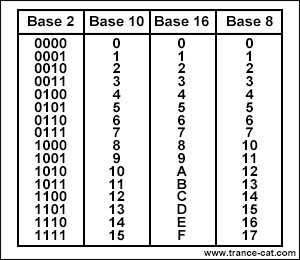# Decimal to Binary, Octal, Hexadecimal Calculator

This calculator is able to calculate the base-2 to 36 numbers of a base-10(decimal) number.

Binary is also known as "base-2" and most machine level computations are done in binary. They are represented as "0"s and "1"s. Logic gates use binary, usually with the ground level voltage representing 0 and a positive voltage representing 1.

Octal also known as "base-8" represents values with the numerals 0 to 7.

Decimal also known as "base-10" is the most widely used numerical base, and is expressed with the numerals 0 to 9.

Hexadecimal also known as "base-16" is commonly used in applications like CSS and Photoshop to represent colors. For example, "FFF" is used to refer to the color white. In this case, where the colors are represented in 3 digits, the first character signals the strength of the color red, whereas the second and third represent the colors green and blue respectively. The colors red, green, and blue make the color white through additive color mixing. Since the strengths of the colors are all the maximum hexadecimal value, FFF is the color, white.

Base-36 represents values with the numerals 0 to 9 and all the characters of the alphabet(A to Z).### Input

Convert (Decimal)
to Base-